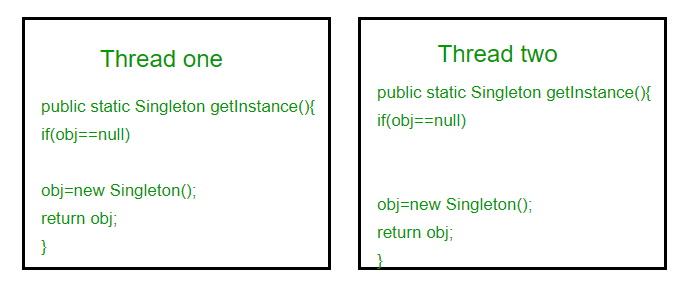# Singleton Design Pattern | Implementation

Singleton Design Pattern | Introduction

The singleton pattern is one of the simplest design patterns. Sometimes we need to have only one instance of our class for example a single DB connection shared by multiple objects as creating a separate DB connection for every object may be costly. Similarly, there can be a single configuration manager or error manager in an application that handles all problems instead of creating multiple managers.

Definition:
The singleton pattern is a design pattern that restricts the instantiation of a class to one object.
Let’s see various design options for implementing such a class. If you have a good handle on static class variables and access modifiers this should not be a difficult task.

Method 1: Classic Implementation

 `// Classical Java implementation of singleton  ` `// design pattern ` `class` `Singleton ` `{ ` `    ``private` `static` `Singleton obj; ` ` `  `    ``// private constructor to force use of ` `    ``// getInstance() to create Singleton object ` `    ``private` `Singleton() {} ` ` `  `    ``public` `static` `Singleton getInstance() ` `    ``{ ` `        ``if` `(obj==``null``) ` `            ``obj = ``new` `Singleton(); ` `        ``return` `obj; ` `    ``} ` `} `

Here we have declared getInstance() static so that we can call it without instantiating the class. The first time getInstance() is called it creates a new singleton object and after that it just returns the same object. Note that Singleton obj is not created until we need it and call getInstance() method. This is called lazy instantiation.

The main problem with above method is that it is not thread safe. Consider the following execution sequence.This execution sequence creates two objects for singleton. Therefore this classic implementation is not thread safe.

Method 2: make getInstance() synchronized

 `// Thread Synchronized Java implementation of  ` `// singleton design pattern ` `class` `Singleton ` `{ ` `    ``private` `static` `Singleton obj; ` ` `  `    ``private` `Singleton() {} ` ` `  `    ``// Only one thread can execute this at a time ` `    ``public` `static` `synchronized` `Singleton getInstance() ` `    ``{ ` `        ``if` `(obj==``null``) ` `            ``obj = ``new` `Singleton(); ` `        ``return` `obj; ` `    ``} ` `} `

Here using synchronized makes sure that only one thread at a time can execute getInstance().
The main disadvantage of this is method is that using synchronized every time while creating the singleton object is expensive and may decrease the performance of your program. However if performance of getInstance() is not critical for your application this method provides a clean and simple solution.

Method 3: Eager Instantiation

 `// Static initializer based Java implementation of ` `// singleton design pattern ` `class` `Singleton ` `{ ` `    ``private` `static` `Singleton obj = ``new` `Singleton(); ` ` `  `    ``private` `Singleton() {} ` ` `  `    ``public` `static` `Singleton getInstance() ` `    ``{ ` `        ``return` `obj; ` `    ``} ` `} `

Here we have created instance of singleton in static initializer. JVM executes static initializer when the class is loaded and hence this is guaranteed to be thread safe. Use this method only when your singleton class is light and is used throughout the execution of your program.

Method 4 (Best): Use “Double Checked Locking

If you notice carefully once an object is created synchronization is no longer useful because now obj will not be null and any sequence of operations will lead to consistent results.
So we will only acquire lock on the getInstance() once, when the obj is null. This way we only synchronize the first way through, just what we want.

 `// Double Checked Locking based Java implementation of ` `// singleton design pattern ` `class` `Singleton ` `{ ` `    ``private` `volatile` `static` `Singleton obj; ` ` `  `    ``private` `Singleton() {} ` ` `  `    ``public` `static` `Singleton getInstance() ` `    ``{ ` `        ``if` `(obj == ``null``) ` `        ``{ ` `            ``// To make thread safe ` `            ``synchronized` `(Singleton.``class``) ` `            ``{ ` `                ``// check again as multiple threads ` `                ``// can reach above step ` `                ``if` `(obj==``null``) ` `                    ``obj = ``new` `Singleton(); ` `            ``} ` `        ``} ` `        ``return` `obj; ` `    ``} ` `} `

We have declared the obj volatile which ensures that multiple threads offer the obj variable correctly when it is being initialized to Singleton instance. This method drastically reduces the overhead of calling the synchronized method every time.

References:

Head First Design Patterns book (Highly recommended)
https://en.wikipedia.org/wiki/Singleton_pattern